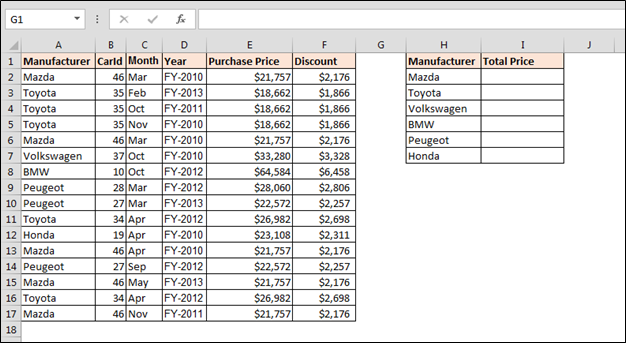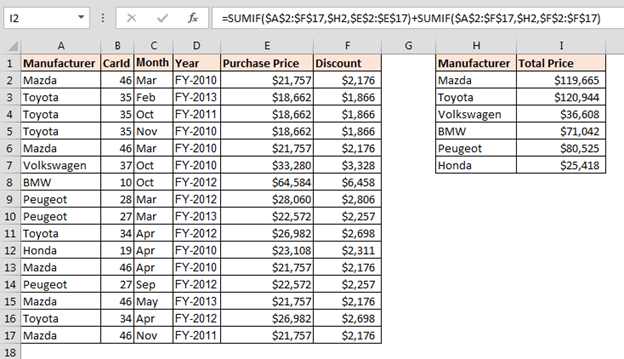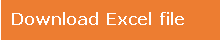# Totaling Two Ranges Using the SUMIF Formula

In this article, we are going to learn how to total two ranges using SUMIF formula in Microsoft Excel.

To let the SUMIF formula add or subtract amounts from different ranges, insert two SUMIF formulas and combine them into a single formula.

Let’s take an example and understand:-

We have an agency’s car sales data. In data, we have sales details along with discount detail. Now, we want to calculate the sum for every manufacturer sales amount and discount amount.How to total two ranges using SUMIF formula?

To total the positive numbers and negative, we will use SUMIF function. We need to follow below given steps:-

• Enter the formula in cell I2
• =SUMIF(\$A\$2:\$F\$17,\$H2,\$E\$2:\$E\$17)+SUMIF(\$A\$2:\$F\$17,\$H2,\$F\$2:\$F\$17)
• Press Enter and copy the same formula in range.

Formula Explanation: -

This syntax “=SUMIF(\$A\$2:\$F\$17,\$H2,\$E\$2:\$E\$17)” will return total sum of purchase amount to every manufacturer.

“SUMIF(\$A\$2:\$F\$17,\$H2,\$F\$2:\$F\$17)” will return total sum of discount amount to every manufacturer.

And then we add the both amount.This is the way we can total two ranges by using SUMIF function in Microsoft Excel.1.i have a problem regarding to how to use sumif with multiple criteria in excel wherein iam having two rows of data and some on the cells do have "NA" in them. Iam using the the formula below but shows error, iam using this formula to add two rows of data (1,2,3 or NA) and dividing by the total number of cells excluding "NA" ones. =IFERROR((SUMIF(F54:F73,M7:M66),"NA")/COUNT((F54:F73,M7:M66),"na"),"")

•Looks like you have missed the criteria in sumif function. only criteria range and sum range is mentioned. SUMIF(F54:F73,M7:M66). Then instead of countif you have use COUNT function.
SUMIF: https://www.exceltip.com/excel-formula-and-function/excel-sumif-function.html
COUNTIF: https://www.exceltip.com/tips/countif-in-microsoft-excel.html

2.Thank a million Allan.

3."I would guess that you are entering the value in A2 in wrongly perhaps?

If you do that, and use the forumula above, then do the following:

1) Enter the formula / data (remember - array formula MUST be Shift-Ctrl-Enter)

2) In the formula bar, highlight G2:G7 ONLY

3) Press F9 and copy / paste whatever G2:G7 changes into back here.

4) Press escape a few times to get back to a normal formula

5) Highlight A2 or Value(A2) whichever you are using in the formula bar.

6) Copy / paste that result here.

We should be able to sort it out then. "

4."Thanks. I keep getting a #Value! unless I write the date in the formula. Referring to a cell, like A2 in yours, returns an error value.

Still, thanks so much for teaching me to get around sumif."

5."You can certainly replace that date (text), or the date value in the formula with a reference.

For example, if you had a cell (A1) that contained the text ""31 July 2003"", then the formula would read:

{=SUM((G2:G7>VALUE(A1))*B2:B7)}

If you had a cell (A2) that contained the value 37833 (the date value for 31 July 2003), then the formula would read:

{=SUM((G2:G7>A2)*B2:B7)}

Are they not working for you in some way? "

6."what do you recommend in lieu of having to write out the date in the formula? Your solution works perfectly, but not if I refer to a cell as opposed to writing out the date (as you have below). Thanks again Alan.

=SUM((G2:G7>VALUE(""31 July 2003""))*B2:B7)"

7."SUMIF is just a dummed down form of an array formula.

I suggest that you never use SUMIF. Partly because once you understand the power of array formulae, there is no need - it is too limiting.

However, a better reason is that *when* someone else picks up your spreadsheet, and doesn't have the right add-in installed, the SUMIF functions all fall to pieces. Better to use native Excel functions, or set them up as UDFs within the file in my opinion.

BTW - Does anyone know whether SUMIF is still part of an add-in with the latest version(s) of Excel? "

8.Thank you so much for this trick about the array functions and this way to use the SUM function ! This is much more powerful than the sumif ! I could add the product of 2 ranges with a condition on a 3rd range !

9."I try to steer clear of SUMIF since it is part an AddIn and not a 'standard' Excel function. That means that if someone who has not installed the Analysis Toolpak (I believe) trys to open your spreadsheet, they will get #NAME errors since the SUMIF function will not be available.

It is not really necessary anyway, since you can easily achieve the same thing with an array formula.

Assuming you have dates in (G2:G7), and related data in (B2:B7) that you want to sum if the date is greater than a given entry:

Enter the following array formula:

=SUM((G2:G7>VALUE(""31 July 2003""))*B2:B7)

Note that if the dates include a time element (non integers) then sometime during the day of 31 July 2003 will be after the date shown above. Therefore you may have to either clean your data or be very explicit about the time that you want to cut off.

You can also use a reference to a date / time value rather than the expression (VALUE(""31 July 2003"")) I used above.

To enter an array formula, type it as shown, then press Ctrl - Shift - Enter together (not just Enter) and Excel will put the braces around it.

Check Excel help for more info on array formulae - very useful to learn! "

10."I am trying to sum data in column B if date in Column G is > than today's date. The formula below works only if your write in the date in the formula, but not if you provide a cell referrence in it (like G4, where G4 is 6/30/2003). Any ideas? Thanks, N

=SUMIF(B:B,G4,D:D)-SUMIF(B:B,G4,E:E)"

Terms and Conditions of use

The applications/code on this site are distributed as is and without warranties or liability. In no event shall the owner of the copyrights, or the authors of the applications/code be liable for any loss of profit, any problems or any damage resulting from the use or evaluation of the applications/code.